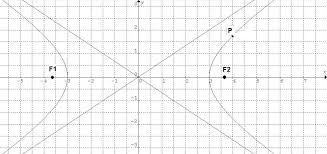## Friday, March 1, 2019

### Solutions To Problems For Analytic Geometry Revisited (2)

The Problems Again:

1)Given  V(-3, 1) and F (0, 1) find the equation of the associated parabola and also for its directrix. Sketch the graph showing the focus, vertex and directrix.

2)  Find the tangent to the parabola y  =   x2     using the fact that the equation of the line tangent to a curve y = f(x) is given by:

y - y1 = f'(x1) (x - x1)

where (x1, y1) = P1 is the point of the curve for which the tangent is constructed and y1  =   (x1)2     .  Show the graph of the given parabola and the tangent line with the point P1 (x1, y1) identified.

3)Analyze the equation below using the translation of axes approach and graph the resulting curve:

x 2   -   4  y 2    -  2 x   + 8 y -  2 = 0

Solutions:

1) From the vertex coordinates we have: h = -3 and k = 1

With these values this indicates a parabola opening to the right  with the general form:

(y -   k)
2  =    4p (x -  h)

applies, so:

(y -   1)
2  =    12 (x  + 3 )

For which 4p  = 12 and p = 3, so we need a directrix equation of form x = -p

The graph is shown below:

2)  We have:  f'(x1)  = dy/ dx =  2x

So:  y - y1 = 2x1  (x - x1)

But only one point satisfies y1  =   (x1)
2

That is x1 = 1, y1 = 1

Hence:

y - 1 = 2 (x -1) or:   y = 2x  - 1

And this tangent line is shown below for the point P1 (x1, y1) = (1,1)3) Collect x and y terms separately:

(x 2   -   2 x)   -   4  (y 2   -  2 y)    =  7

(x 2   -   2 x    +   1)  - 4(y 2   -  2 y   + 1) =  7  + 1  - 4 =  5

Or:

(x  +  1 ) 2 /  4  -   (y  -   1 ) 2    =  1

Let:   x’  =  x +  1,   and:   y’  = y  -  1

Then:   x’  2 /  4   -   y’  2 /  1   =   1

Graphing: